Elementary Algebra

# 6.6Divide Polynomials

Elementary Algebra6.6 Divide Polynomials

### Learning Objectives

By the end of this section, you will be able to:

• Divide a polynomial by a monomial
• Divide a polynomial by a binomial

### Be Prepared 6.6

Before you get started, take this readiness quiz.

1. Add: $3d+xd.3d+xd.$
If you missed this problem, review Example 1.77.
2. Simplify: $30xy35xy.30xy35xy.$
If you missed this problem, review Example 6.72.
3. Combine like terms: $8a2+12a+1+3a2−5a+4.8a2+12a+1+3a2−5a+4.$
If you missed this problem, review Example 1.24.

### Divide a Polynomial by a Monomial

In the last section, you learned how to divide a monomial by a monomial. As you continue to build up your knowledge of polynomials the next procedure is to divide a polynomial of two or more terms by a monomial.

The method we’ll use to divide a polynomial by a monomial is based on the properties of fraction addition. So we’ll start with an example to review fraction addition.

 The sum, $y5+25,y5+25,$ simplifies to $y+25.y+25.$
Table 6.2

Now we will do this in reverse to split a single fraction into separate fractions.

We’ll state the fraction addition property here just as you learned it and in reverse.

If $a,b,andca,b,andc$ are numbers where $c≠0c≠0$, then

$ac+bc=a+bcanda+bc=ac+bcac+bc=a+bcanda+bc=ac+bc$

We use the form on the left to add fractions and we use the form on the right to divide a polynomial by a monomial.

 For example, $y+25y+25$ can be written $y5+25.y5+25.$
Table 6.3

We use this form of fraction addition to divide polynomials by monomials.

### Division of a Polynomial by a Monomial

To divide a polynomial by a monomial, divide each term of the polynomial by the monomial.

### Example 6.77

Find the quotient: $7y2+217.7y2+217.$

### Try It 6.153

Find the quotient: $8z2+244.8z2+244.$

### Try It 6.154

Find the quotient: $18z2−279.18z2−279.$

Remember that division can be represented as a fraction. When you are asked to divide a polynomial by a monomial and it is not already in fraction form, write a fraction with the polynomial in the numerator and the monomial in the denominator.

### Example 6.78

Find the quotient: $(18x3−36x2)÷6x.(18x3−36x2)÷6x.$

### Try It 6.155

Find the quotient: $(27b3−33b2)÷3b.(27b3−33b2)÷3b.$

### Try It 6.156

Find the quotient: $(25y3−55y2)÷5y.(25y3−55y2)÷5y.$

When we divide by a negative, we must be extra careful with the signs.

### Example 6.79

Find the quotient: $12d2−16d−4.12d2−16d−4.$

### Try It 6.157

Find the quotient: $25y2−15y−5.25y2−15y−5.$

### Try It 6.158

Find the quotient: $42b2−18b−6.42b2−18b−6.$

### Example 6.80

Find the quotient: $105y5+75y35y2.105y5+75y35y2.$

### Try It 6.159

Find the quotient: $60d7+24d54d3.60d7+24d54d3.$

### Try It 6.160

Find the quotient: $216p7−48p56p3.216p7−48p56p3.$

### Example 6.81

Find the quotient: $(15x3y−35xy2)÷(−5xy).(15x3y−35xy2)÷(−5xy).$

### Try It 6.161

Find the quotient: $(32a2b−16ab2)÷(−8ab).(32a2b−16ab2)÷(−8ab).$

### Try It 6.162

Find the quotient: $(−48a8b4−36a6b5)÷(−6a3b3).(−48a8b4−36a6b5)÷(−6a3b3).$

### Example 6.82

Find the quotient: $36x3y2+27x2y2−9x2y39x2y.36x3y2+27x2y2−9x2y39x2y.$

### Try It 6.163

Find the quotient: $40x3y2+24x2y2−16x2y38x2y.40x3y2+24x2y2−16x2y38x2y.$

### Try It 6.164

Find the quotient: $35a4b2+14a4b3−42a2b47a2b2.35a4b2+14a4b3−42a2b47a2b2.$

### Example 6.83

Find the quotient: $10x2+5x−205x.10x2+5x−205x.$

### Try It 6.165

Find the quotient: $18c2+6c−96c.18c2+6c−96c.$

### Try It 6.166

Find the quotient: $10d2−5d−25d.10d2−5d−25d.$

### Divide a Polynomial by a Binomial

To divide a polynomial by a binomial, we follow a procedure very similar to long division of numbers. So let’s look carefully the steps we take when we divide a 3-digit number, 875, by a 2-digit number, 25.

 We write the long division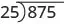We divide the first two digits, 87, by 25.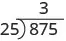We multiply 3 times 25 and write the product under the 87.Now we subtract 75 from 87.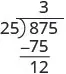Then we bring down the third digit of the dividend, 5.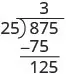Repeat the process, dividing 25 into 125.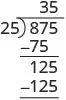We check division by multiplying the quotient by the divisor.

If we did the division correctly, the product should equal the dividend.

$35·25875✓35·25875✓$

Now we will divide a trinomial by a binomial. As you read through the example, notice how similar the steps are to the numerical example above.

### Example 6.84

Find the quotient: $(x2+9x+20)÷(x+5).(x2+9x+20)÷(x+5).$

### Try It 6.167

Find the quotient: $(y2+10y+21)÷(y+3).(y2+10y+21)÷(y+3).$

### Try It 6.168

Find the quotient: $(m2+9m+20)÷(m+4).(m2+9m+20)÷(m+4).$

When the divisor has subtraction sign, we must be extra careful when we multiply the partial quotient and then subtract. It may be safer to show that we change the signs and then add.

### Example 6.85

Find the quotient: $(2x2−5x−3)÷(x−3).(2x2−5x−3)÷(x−3).$

### Try It 6.169

Find the quotient: $(2x2−3x−20)÷(x−4).(2x2−3x−20)÷(x−4).$

### Try It 6.170

Find the quotient: $(3x2−16x−12)÷(x−6).(3x2−16x−12)÷(x−6).$

When we divided 875 by 25, we had no remainder. But sometimes division of numbers does leave a remainder. The same is true when we divide polynomials. In Example 6.86, we’ll have a division that leaves a remainder. We write the remainder as a fraction with the divisor as the denominator.

### Example 6.86

Find the quotient: $(x3−x2+x+4)÷(x+1).(x3−x2+x+4)÷(x+1).$

### Try It 6.171

Find the quotient: $(x3+5x2+8x+6)÷(x+2).(x3+5x2+8x+6)÷(x+2).$

### Try It 6.172

Find the quotient: $(2x3+8x2+x−8)÷(x+1).(2x3+8x2+x−8)÷(x+1).$

Look back at the dividends in Example 6.84, Example 6.85, and Example 6.86. The terms were written in descending order of degrees, and there were no missing degrees. The dividend in Example 6.87 will be $x4−x2+5x−2x4−x2+5x−2$. It is missing an $x3x3$ term. We will add in $0x30x3$ as a placeholder.

### Example 6.87

Find the quotient: $(x4−x2+5x−2)÷(x+2).(x4−x2+5x−2)÷(x+2).$

### Try It 6.173

Find the quotient: $(x3+3x+14)÷(x+2).(x3+3x+14)÷(x+2).$

### Try It 6.174

Find the quotient: $(x4−3x3−1000)÷(x+5).(x4−3x3−1000)÷(x+5).$

In Example 6.88, we will divide by $2a−32a−3$. As we divide we will have to consider the constants as well as the variables.

### Example 6.88

Find the quotient: $(8a3+27)÷(2a+3).(8a3+27)÷(2a+3).$

### Try It 6.175

Find the quotient: $(x3−64)÷(x−4).(x3−64)÷(x−4).$

### Try It 6.176

Find the quotient: $(125x3−8)÷(5x−2).(125x3−8)÷(5x−2).$

### Media

Access these online resources for additional instruction and practice with dividing polynomials:

### Section 6.6 Exercises

#### Practice Makes Perfect

In the following exercises, divide each polynomial by the monomial.

442.

$45 y + 36 9 45 y + 36 9$

443.

$30 b + 75 5 30 b + 75 5$

444.

$8 d 2 − 4 d 2 8 d 2 − 4 d 2$

445.

$42 x 2 − 14 x 7 42 x 2 − 14 x 7$

446.

$( 16 y 2 − 20 y ) ÷ 4 y ( 16 y 2 − 20 y ) ÷ 4 y$

447.

$( 55 w 2 − 10 w ) ÷ 5 w ( 55 w 2 − 10 w ) ÷ 5 w$

448.

$( 9 n 4 + 6 n 3 ) ÷ 3 n ( 9 n 4 + 6 n 3 ) ÷ 3 n$

449.

$( 8 x 3 + 6 x 2 ) ÷ 2 x ( 8 x 3 + 6 x 2 ) ÷ 2 x$

450.

$18 y 2 − 12 y −6 18 y 2 − 12 y −6$

451.

$20 b 2 − 12 b −4 20 b 2 − 12 b −4$

452.

$35 a 4 + 65 a 2 −5 35 a 4 + 65 a 2 −5$

453.

$51 m 4 + 72 m 3 −3 51 m 4 + 72 m 3 −3$

454.

$310 y 4 − 200 y 3 5 y 2 310 y 4 − 200 y 3 5 y 2$

455.

$412 z 8 − 48 z 5 4 z 3 412 z 8 − 48 z 5 4 z 3$

456.

$46 x 3 + 38 x 2 2 x 2 46 x 3 + 38 x 2 2 x 2$

457.

$51 y 4 + 42 y 2 3 y 2 51 y 4 + 42 y 2 3 y 2$

458.

$( 24 p 2 − 33 p ) ÷ ( −3 p ) ( 24 p 2 − 33 p ) ÷ ( −3 p )$

459.

$( 35 x 4 − 21 x ) ÷ ( −7 x ) ( 35 x 4 − 21 x ) ÷ ( −7 x )$

460.

$( 63 m 4 − 42 m 3 ) ÷ ( −7 m 2 ) ( 63 m 4 − 42 m 3 ) ÷ ( −7 m 2 )$

461.

$( 48 y 4 − 24 y 3 ) ÷ ( −8 y 2 ) ( 48 y 4 − 24 y 3 ) ÷ ( −8 y 2 )$

462.

$( 63 a 2 b 3 + 72 a b 4 ) ÷ ( 9 a b ) ( 63 a 2 b 3 + 72 a b 4 ) ÷ ( 9 a b )$

463.

$( 45 x 3 y 4 + 60 x y 2 ) ÷ ( 5 x y ) ( 45 x 3 y 4 + 60 x y 2 ) ÷ ( 5 x y )$

464.

$52 p 5 q 4 + 36 p 4 q 3 − 64 p 3 q 2 4 p 2 q 52 p 5 q 4 + 36 p 4 q 3 − 64 p 3 q 2 4 p 2 q$

465.

$49 c 2 d 2 − 70 c 3 d 3 − 35 c 2 d 4 7 c d 2 49 c 2 d 2 − 70 c 3 d 3 − 35 c 2 d 4 7 c d 2$

466.

$66 x 3 y 2 − 110 x 2 y 3 − 44 x 4 y 3 11 x 2 y 2 66 x 3 y 2 − 110 x 2 y 3 − 44 x 4 y 3 11 x 2 y 2$

467.

$72 r 5 s 2 + 132 r 4 s 3 − 96 r 3 s 5 12 r 2 s 2 72 r 5 s 2 + 132 r 4 s 3 − 96 r 3 s 5 12 r 2 s 2$

468.

$4 w 2 + 2 w − 5 2 w 4 w 2 + 2 w − 5 2 w$

469.

$12 q 2 + 3 q − 1 3 q 12 q 2 + 3 q − 1 3 q$

470.

$10 x 2 + 5 x − 4 −5 x 10 x 2 + 5 x − 4 −5 x$

471.

$20 y 2 + 12 y − 1 −4 y 20 y 2 + 12 y − 1 −4 y$

472.

$36 p 3 + 18 p 2 − 12 p 6 p 2 36 p 3 + 18 p 2 − 12 p 6 p 2$

473.

$63 a 3 − 108 a 2 + 99 a 9 a 2 63 a 3 − 108 a 2 + 99 a 9 a 2$

Divide a Polynomial by a Binomial

In the following exercises, divide each polynomial by the binomial.

474.

$( y 2 + 7 y + 12 ) ÷ ( y + 3 ) ( y 2 + 7 y + 12 ) ÷ ( y + 3 )$

475.

$( d 2 + 8 d + 12 ) ÷ ( d + 2 ) ( d 2 + 8 d + 12 ) ÷ ( d + 2 )$

476.

$( x 2 − 3 x − 10 ) ÷ ( x + 2 ) ( x 2 − 3 x − 10 ) ÷ ( x + 2 )$

477.

$( a 2 − 2 a − 35 ) ÷ ( a + 5 ) ( a 2 − 2 a − 35 ) ÷ ( a + 5 )$

478.

$( t 2 − 12 t + 36 ) ÷ ( t − 6 ) ( t 2 − 12 t + 36 ) ÷ ( t − 6 )$

479.

$( x 2 − 14 x + 49 ) ÷ ( x − 7 ) ( x 2 − 14 x + 49 ) ÷ ( x − 7 )$

480.

$( 6 m 2 − 19 m − 20 ) ÷ ( m − 4 ) ( 6 m 2 − 19 m − 20 ) ÷ ( m − 4 )$

481.

$( 4 x 2 − 17 x − 15 ) ÷ ( x − 5 ) ( 4 x 2 − 17 x − 15 ) ÷ ( x − 5 )$

482.

$( q 2 + 2 q + 20 ) ÷ ( q + 6 ) ( q 2 + 2 q + 20 ) ÷ ( q + 6 )$

483.

$( p 2 + 11 p + 16 ) ÷ ( p + 8 ) ( p 2 + 11 p + 16 ) ÷ ( p + 8 )$

484.

$( y 2 − 3 y − 15 ) ÷ ( y − 8 ) ( y 2 − 3 y − 15 ) ÷ ( y − 8 )$

485.

$( x 2 + 2 x − 30 ) ÷ ( x − 5 ) ( x 2 + 2 x − 30 ) ÷ ( x − 5 )$

486.

$( 3 b 3 + b 2 + 2 ) ÷ ( b + 1 ) ( 3 b 3 + b 2 + 2 ) ÷ ( b + 1 )$

487.

$( 2 n 3 − 10 n + 24 ) ÷ ( n + 3 ) ( 2 n 3 − 10 n + 24 ) ÷ ( n + 3 )$

488.

$( 2 y 3 − 6 y − 36 ) ÷ ( y − 3 ) ( 2 y 3 − 6 y − 36 ) ÷ ( y − 3 )$

489.

$( 7 q 3 − 5 q − 2 ) ÷ ( q − 1 ) ( 7 q 3 − 5 q − 2 ) ÷ ( q − 1 )$

490.

$( z 3 + 1 ) ÷ ( z + 1 ) ( z 3 + 1 ) ÷ ( z + 1 )$

491.

$( m 3 + 1000 ) ÷ ( m + 10 ) ( m 3 + 1000 ) ÷ ( m + 10 )$

492.

$( a 3 − 125 ) ÷ ( a − 5 ) ( a 3 − 125 ) ÷ ( a − 5 )$

493.

$( x 3 − 216 ) ÷ ( x − 6 ) ( x 3 − 216 ) ÷ ( x − 6 )$

494.

$( 64 x 3 − 27 ) ÷ ( 4 x − 3 ) ( 64 x 3 − 27 ) ÷ ( 4 x − 3 )$

495.

$( 125 y 3 − 64 ) ÷ ( 5 y − 4 ) ( 125 y 3 − 64 ) ÷ ( 5 y − 4 )$

#### Everyday Math

496.

Average cost Pictures Plus produces digital albums. The company’s average cost (in dollars) to make $xx$ albums is given by the expression $7x+500x7x+500x$.

1. Find the quotient by dividing the numerator by the denominator.
2. What will the average cost (in dollars) be to produce 20 albums?
497.

Handshakes At a company meeting, every employee shakes hands with every other employee. The number of handshakes is given by the expression $n2−n2n2−n2$, where $nn$ represents the number of employees. How many handshakes will there be if there are 10 employees at the meeting?

#### Writing Exercises

498.

James divides $48y+648y+6$ by 6 this way: $48y+66=48y48y+66=48y$. What is wrong with his reasoning?

499.

Divide $10x2+x−122x10x2+x−122x$ and explain with words how you get each term of the quotient.

#### Self Check

After completing the exercises, use this checklist to evaluate your mastery of the objectives of this section.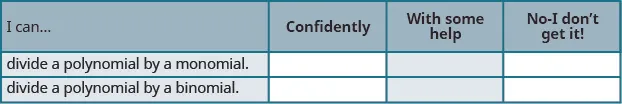After reviewing this checklist, what will you do to become confident for all goals?

Order a print copy

As an Amazon Associate we earn from qualifying purchases.